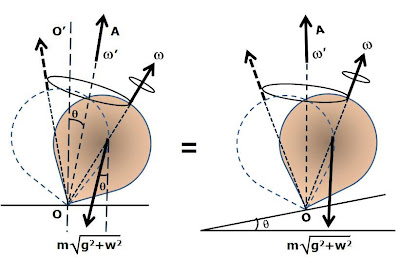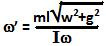## Sunday, March 29, 2009

### Irodov Problem 1.285The easiest way to solve this problem is using Einstein's famous principle of equivalence. Since, the entire top moves with a linear acceleration w in the horizontal direction, it is as if it experiences a net force of gravity equivalent toin a direction tilted to the vertical at an angle.

Now all we have to do is to solve this problem exactly as 1.283, with the caveat that gravity is now tilted at an angle and has a different magnitude.Thus unlike in problem 1.283, where the top precessed symmetrically about the vertical axis, in this case, the top will precess symmetrically about the tilted axis OA oriented as shown in the figure. Alternatively, one could imagine that the top itself is on an inclined plane inclined at an angleand on a planet with higher gravity of valueas shown in the figure. Thus, solving the problem exactly as in 1.283 it is clear that,and acts at an angle oftilted to the vertical as shown in the figure.